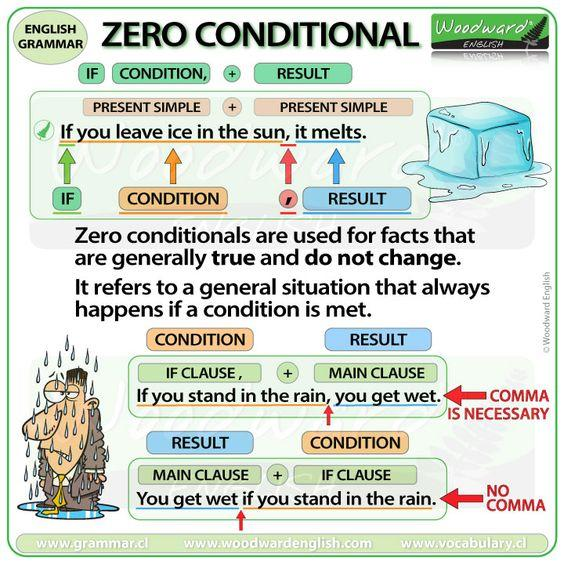STARTBODY

# Zero Conditional Explanation

Conditionals are a fundamental part of English grammar that every teacher should know like the back of their hand. Let's take a look at the zero conditional below.## What is the Zero Conditional?

Zero conditionals are used for facts that are generally true and do not change. They refer to a general situation that always happen (for example in the rules of a game or laws of physics) if a condition is met. The condition always has the same result.

The zero conditional uses the present simple in the if clause and in the main clause.

Zero conditionals are also called "Type 0 conditionals" (general truth – general rule).

### If + condition, result

Let’s take a look at some example sentences:

• If you leave ice in the sun, it melts.

Here, the condition is leaving ice in the sun. This is an if-clause because it begins with the word "if".

What is the result of this if-clause?

The result is that the ice melts.

This is a zero conditional because the condition (if you leave ice in the sun) always has the same result (the ice melts). That is why we used the zero conditional with the if-clause, or condition in the present simple tense and the result in the present simple tense.

### Result + if + condition

You can also change the order of the sentence and place the main clause (the result) before the if-clause (the condition), for example:

• Ice melts if you leave it in the sun.

### IF / WHEN

We can often use WHEN instead of IF and it has the same meaning.

• If you keep milk in the fridge, it lasts longer.
• When you keep milk in the fridge, it lasts longer.

-> The result is always the same. One thing (a condition) always leads to another (a result).

;
ENDBODY